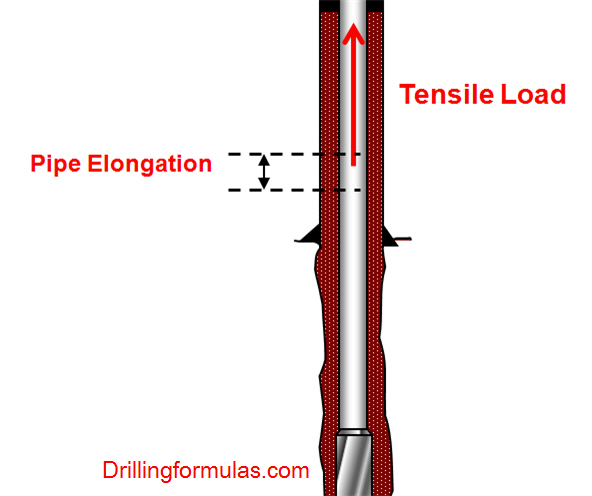## Drillstring Elongation Due To a Tensile Load

When a tensile load is applied into a drillstring, it results in elongation of the drillstring.The formula to determine pipe elongation is listed below;

Oilfield unit

e = (L x T) ÷ (735,000 x W)

Where;

e = elongation, inch

L = length of drill string, ft

W = drill string weight, lb/ft

Metric (SI) unit

e = (L x T) ÷ (26.7 x W)

Where;

e = elongation, mm

L = length of drill string, metres

W = drill string weight, kg/m

Note: The equations are taken from API RP 7G 16th Edition, August 1998.

## System Constrain for Drilling Hydraulics

When we get the optimum flow rate which match your optimization objective, you must check the system constrains such as

• Deliverability of mud pumps is enough, isn’t it?

• The optimum flow rate is within the operating range of down hole tools, isn’t it?

• The maximum annular velocity is exceeded, isn’t it?

• The equivalent circulating density can cause formation fracture, can’t it?

• The flow rate is below the critical flow rate, isn’t it?

In the following articles, we will have more in depth details regarding system constrains for drilling hydraulics. The topics that we will discuss are as follows:

• Annular velocity and its importance on drilling hydraulics

• Power Law constant determination

• Effective viscosity

• Reynolds number

• Flow regime and critical Reynolds numbers

• Critical flow rate

We also have an intention to demonstrate step-by-step calculation to show you all calculation because only equations are not good enough to get all the pictures.

Reference:Drilling Hydraulic Books